# Advanced Bayesian regression in JASP

Greetings!

This tutorial illustrates how to interpret the more advanced output and to set different prior specifications in performing Bayesian regression analyses in JASP (JASP Team, 2020). We explain various options in the control panel and introduce such concepts as Bayesian model averaging, posterior model probability, prior model probability, inclusion Bayes factor, and posterior exclusion probability. After the tutorial, we expect readers can deeply comprehend the Bayesian regression and perform it to answer substantive research questions.

For readers who need fundamentals of JASP, we recommend reading JASP for beginners. If readers need nuts and bolts of Bayesian analyses in JASP, we suggest following JASP for Bayesian analyses with default priors. The current tutorial assumes that readers are equipped with the knowledge necessary for advanced Bayesian regression analysis.

Since we continuously improve the tutorials, let us know if you discover mistakes, or if you have additional resources we can refer to. If you want to be the first to be informed about updates, follow Rens on Twitter.

Let’s get started!

### How to cite this tutorial in APA style

Heo, I., & Van de Schoot, R. (2020, September 14). Advanced Bayesian regression in JASP. Zenodo. https://doi.org/10.5281/zenodo.3991326

## PhD-Delays Dataset

We will use a phd-delay dataset (Van de Schoot, 2020) from Van de Schoot, Yerkes, Mouw, and Sonneveld (2013). To download the dataset, click here.

The dataset is based on a study that asks Ph.D. recipients how long it took them to complete their Ph.D. projects (n = 333). It turned out that the Ph.D. recipients took an average of 59.8 months to finish their Ph.D. trajectory.

For more information on the sample, instruments, methodology, and research context, we refer interested readers to the paper (see references). A brief description of the variables in the dataset follows. The variable names in the table below will be used in the tutorial, henceforth.

Variable name Description
B3_difference_extra The difference between planned and actual project time in months
E4_having_child Whether there are any children under the age of 18 living in the household (0 = no, 1 = yes)
E21_sex Respondents’ gender (0 = female, 1 = male)
E22_Age Respondents’ age
E22_Age_Squared Respondents’ age-squared

### How to cite the dataset in APA style

Van de Schoot, R. (2020). PhD-delay Dataset for Online Stats Training [Data set]. Zenodo. https://doi.org/10.5281/zenodo.3999424

## I. Setting a goal: A research question

• Earning a Ph.D. degree is a long and enduring process. Many factors are involved in timely completion, abrupt termination, or some delay of the Ph.D. life. For the current tutorial, we examine how age is related to the Ph.D. delay.
• Let’s think about the relationship between age and Ph.D. delay. Do you think they are linearly related? Are there any different ideas such that the age is non-linearly associated with the delay? We expect the relationship between the completion time and age are non-linear. This might be because, at a certain point in your life (say mid-thirties), family life takes up more of your time compared to when you are in the twenties.
• To that end, we examine whether the age of the Ph.D. recipients predicts a delay in their Ph.D. projects. The regression model is consequently the one we should adopt to answer the research question. We use the difference variable (B3_difference_extra) as the dependent variable and the age (E22_Age) and the age-squared (E22_Age_Squared) as the independent variables for the regression model.

## II. Eyes on the dataset

• The statistical analysis goes hand in hand with data. If you have not yet downloaded the dataset for our tutorial, click here.
• JASP offers three ways to load the data with simple mouse clicks: from your computer, the in-built data library, or the Open Science Framework.
• If you are not familiar with loading the data, please go to JASP for beginners and see how to load the data.
• It’s time to meet the data in JASP. Did you successfully load your dataset? The column names should correspond to the names of the five variables in the dataset.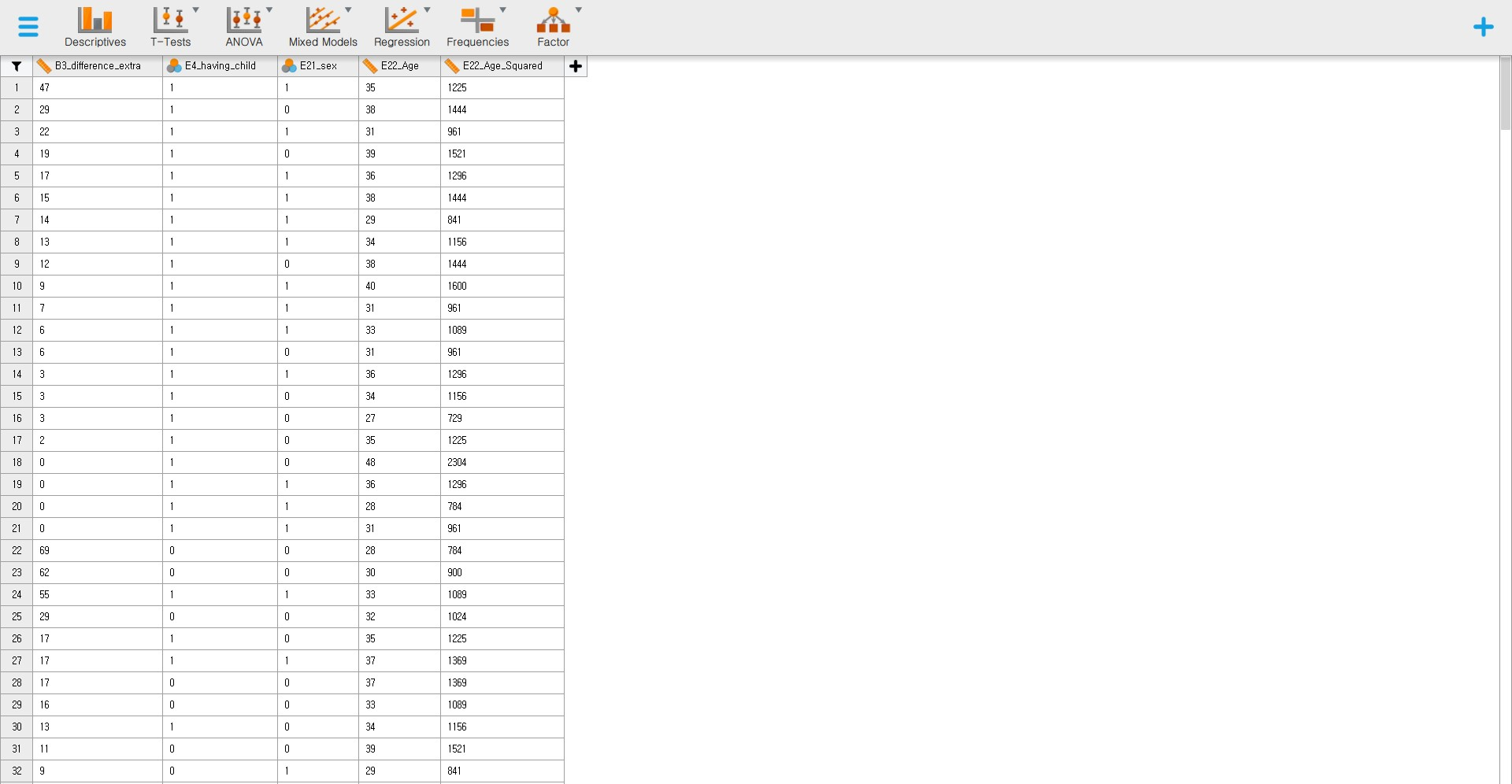### 2. Exploring and inspecting data

• It is always desirable to explore your data once loaded. We can inspect via either descriptive statistics or data visualization.
• If you are not familiar with data exploration, please go to JASP for beginners and check how to inspect the data.

#### Descriptive statistics

• Let’s see the descriptive statistics of the variables to check whether all the data points make sense.
• Click Descriptives at the top bar to proceed with -> Move all the variables to space under the Variables section -> Check Frequency tables (nominal and ordinal variables) -> Click Statistics -> Check additional descriptive statistics (S. E. mean under Dispersion, Median under Central Tendency, and Skewness and Kurtosis under Distribution) for better understanding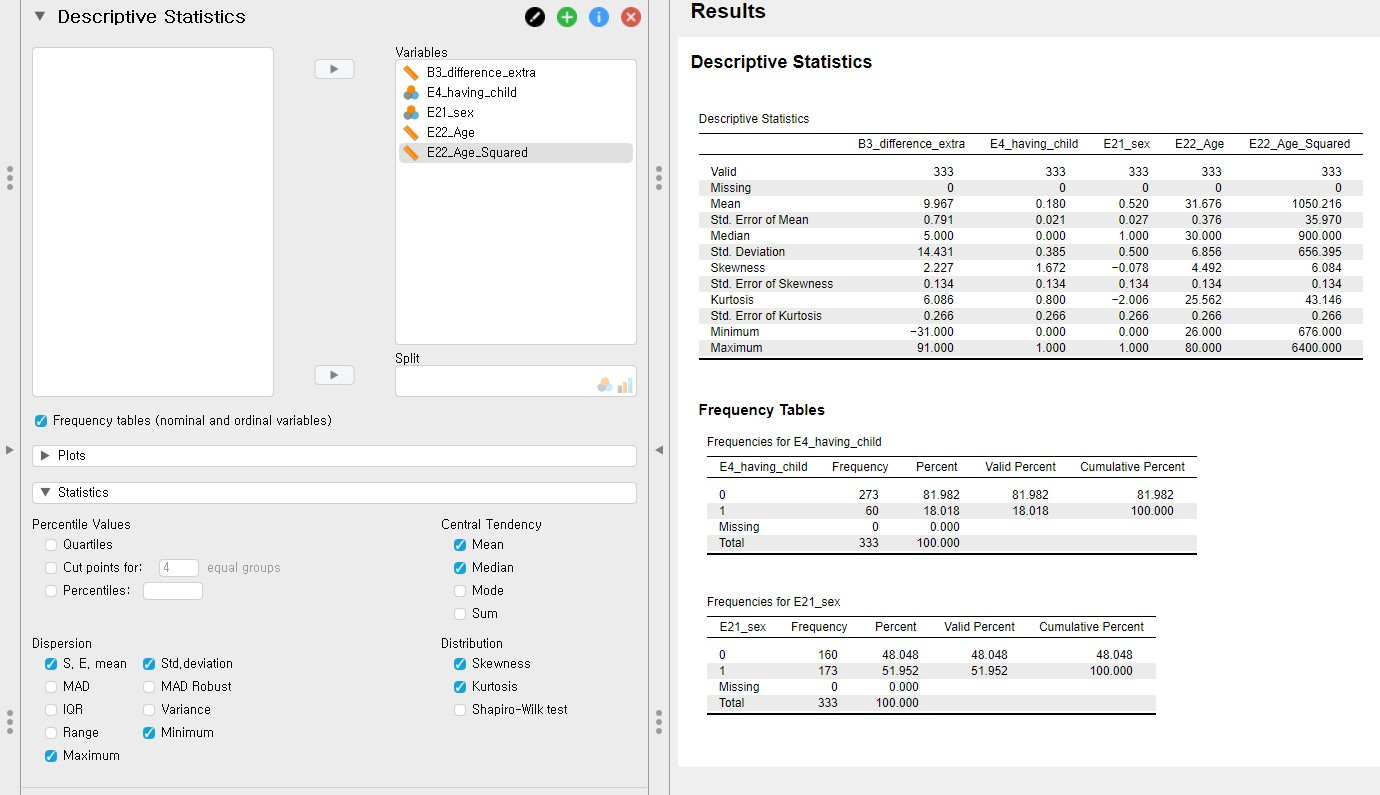• For all the variables, the sample size is 333 without any missing values.
• For the difference variable (B3_difference_extra), the mean and the standard error of the mean are 9.97 and 0.79.
• For the age variable (E22_Age), the mean and the standard error of the mean are 31.68 and 0.38.
• For the age-squared variable (E22_Age_Squared), the mean and the standard error of the mean are 1050.22 and 35.97.
• For the dichotomous variables (E4_having_child and E21_sex), frequency tables are presented.
• Given the descriptive statistics, all the data points substantively make sense.

#### Data visualization

• Let’s plot the expected relationship between age and the delay for visual inspection. To do that, we need to remain only two variables, B3_difference_extra and E22_Age, under the Variables section.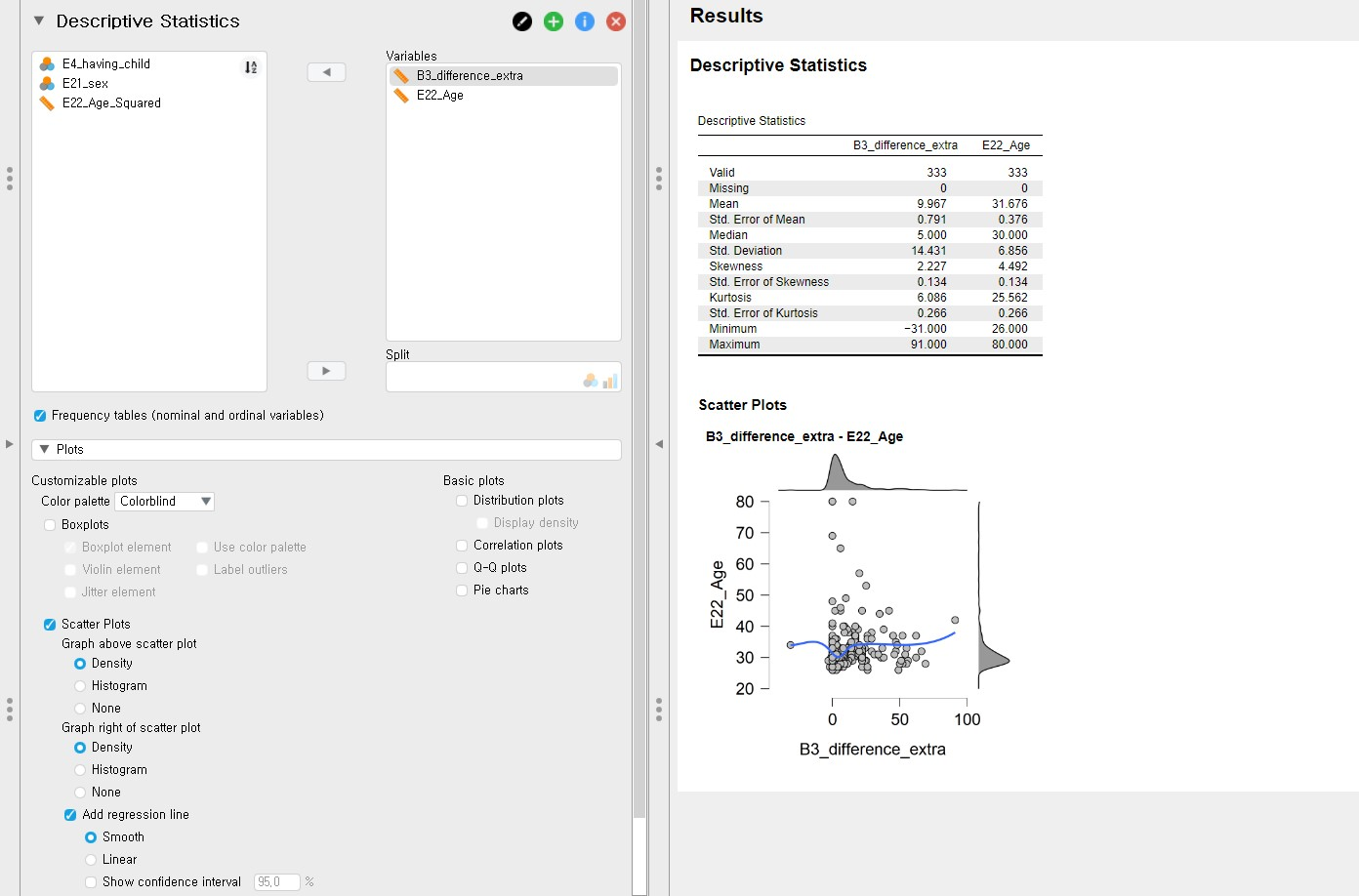• Click Plots and check Scatter Plots -> Under Scatter Plots, uncheck Show confidence interval 95.0%. We can see the scatter plot between the difference variable on the x-axis and the age variable on the y-axis.
• Although we can use this scatter plot for our visual inspection purposes, it might be better to locate the difference variable on the y-axis and the age variable on the x-axis since we regress the difference variable on the age variable. We can simply do that by changing the variable order under the Variables section: set E22_Age to be the first and B3_difference_extra the latter.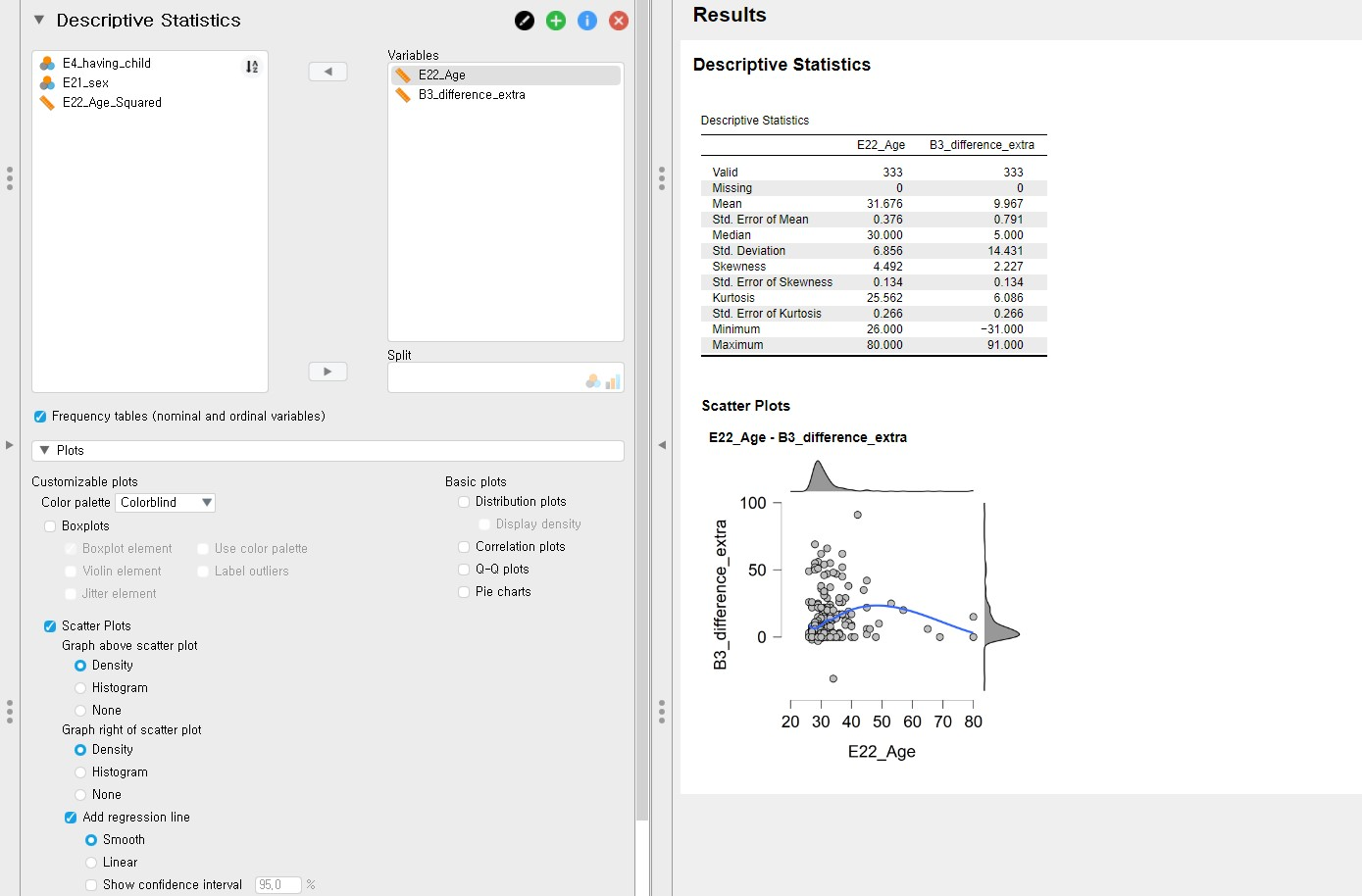• With this plot, we can see the non-linear relationship between the difference variable and the age variable.
• It is also possible to examine the linear relationship by drawing the linear regression line. How? Uncheck Smooth and check Linear under Add regression line. Can you see the linear line?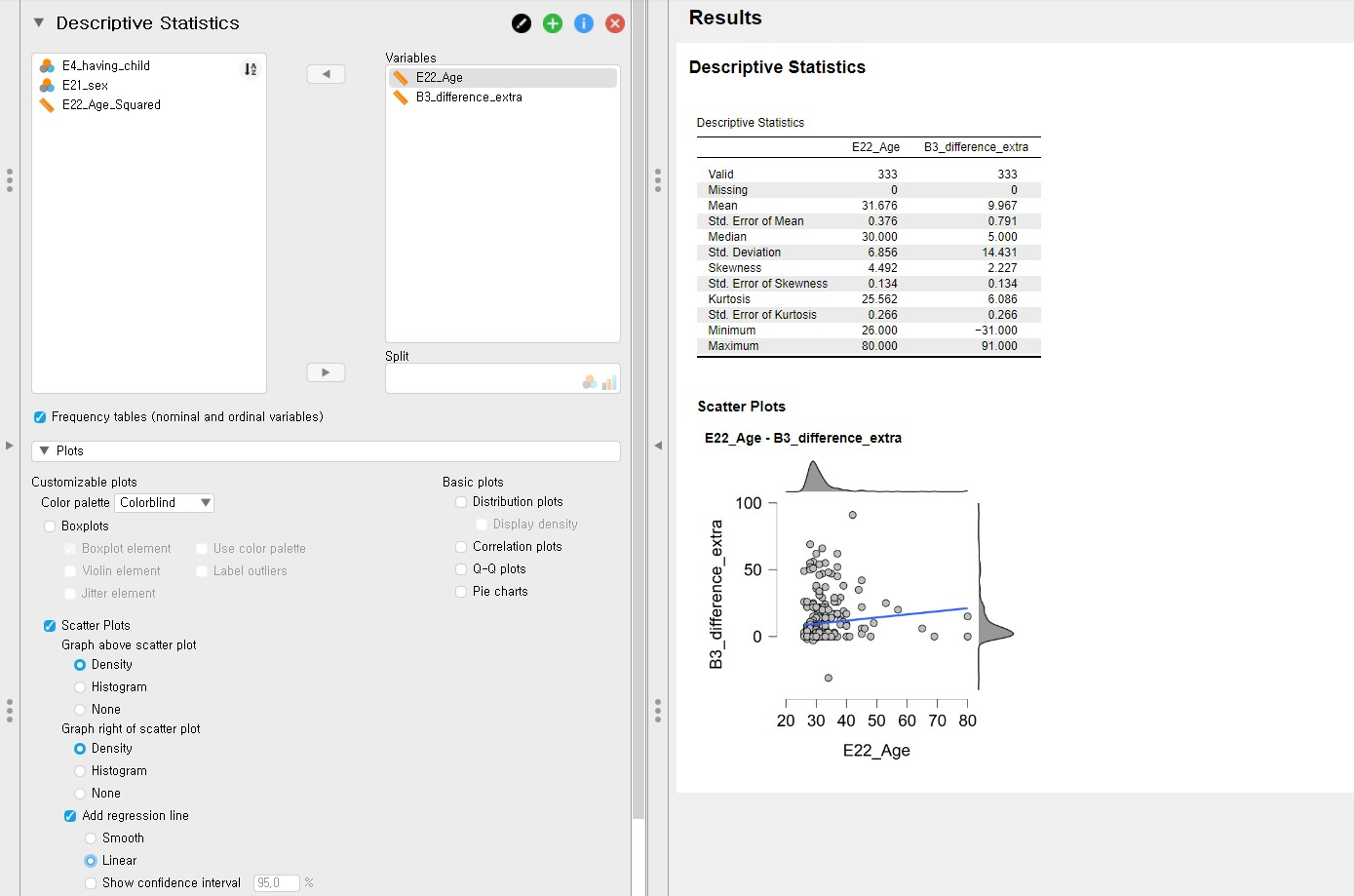## III. Intermezzo: Parameter estimation and hypothesis testing from a Bayesian perspective

### 1. Bayesian parameter estimation

• A notable feature of Bayesian statistics is that the prior distributions of parameters are combined with the likelihood of data to update the prior distributions to the posterior distributions (see Van de Schoot et al., 2014 for introduction and application of Bayesian analysis). The updated posterior distributions are materials for Bayesian statistical inference. This logic implies the crucial role of prior distributions in doing Bayesian statistics!
• In our regression model, parameters are the intercept, two regression coefficients, and residual variance. Thus, we should specify the prior distributions for the intercept, two regression coefficients, and residual variance, respectively.
• Please note that we leave the discussion about priors for the intercept and the residual variance untouched in this exercise. We thus consider the prior distributions for the regression coefficients only.
• Let’s think about the model under consideration to see what the regression coefficients are. The regression formula for the current example can be written as: $$Difference = b_{0} + b_{Age} * X_{Age} + b_{Age-squared} * X_{Age-squared}$$
• You can see that the regression coefficients are $$b_{Age}$$ and $$b_{Age-squared}$$ whereas $$b_{0}$$ is the intercept.
• The regression coefficients you will see in the output panel are the summaries of the posterior distributions of these two regression coefficients.

### 2. Bayesian hypothesis testing

• Bayesian hypothesis testing focuses on which hypothesis receives relatively more support from the observed data. This is quantified by the Bayes factor (Kass & Raftery, 1995).
• Say there are two hypotheses under consideration: the null hypothesis and the alternative hypothesis. If the Bayes factor in favor of the alternative hypothesis is 15, this means that the support in the observed data is about fifteen times larger for the alternative hypothesis than for the null hypothesis. As you can see, how likely the data are to be observed among competing hypotheses is expressed in terms of the Bayes factor.
• Please note that we do not use such words as significant and p-value in Bayesian hypothesis testing. They are the words used under the frequentist framework.

### 3. Setting seed values for reproducible results

• Bayesian inference is based on the posterior distribution of parameters after taking into account the likelihood of data and the prior distribution. The likelihood and the prior are expressed in terms of mathematical functions.
• The potential problem that could arise is that, usually, the posterior distribution is hard to get analytically (see Chapter 5 and Chapter 6 in Kruschke, 2014 for information about conjugate and non-conjugate prior). To solve this issue, Bayesian statistics uses the random number generator to approximate the posterior distribution. Due to generating random numbers, results from Bayesian analyses are slightly different each time we run Bayesian analyses.
• Surprisingly, this is not completely random such that each software has its hidden rule that the sequence of numbers is generated! We can thus say the software is based on a pseudorandom number generator. The idea is that, whenever a certain number is fed as an input to the generator, the software produces the same sequence of pseudorandom numbers. Therefore, researchers and statisticians feed a specific number to the statistical software to make it reproduce the same outputs. This is why we set seed to get reproducible results.
• For more information about setting seed values for Bayesian statistics, see Chapter 7 in Kruschke (2014).

## IV. Doing Bayesian regression analyses with default priors

• We assume that all the assumptions required for subsequent analyses are satisfied.

### 1. In-depth interpretations of the Bayesian regression results

#### A review for interpreting the posterior summary for default priors settings

• For reproducible results, we will set a seed of 123.
• Move B3_difference_extra under the Dependent Variable section and E22_Age and E22_Age_Squared under the Covariates section. Check the Posterior summary under Output in the control panel. Please note that we will use the Model averaged option instead of the Best model option under the Output section in the control panel.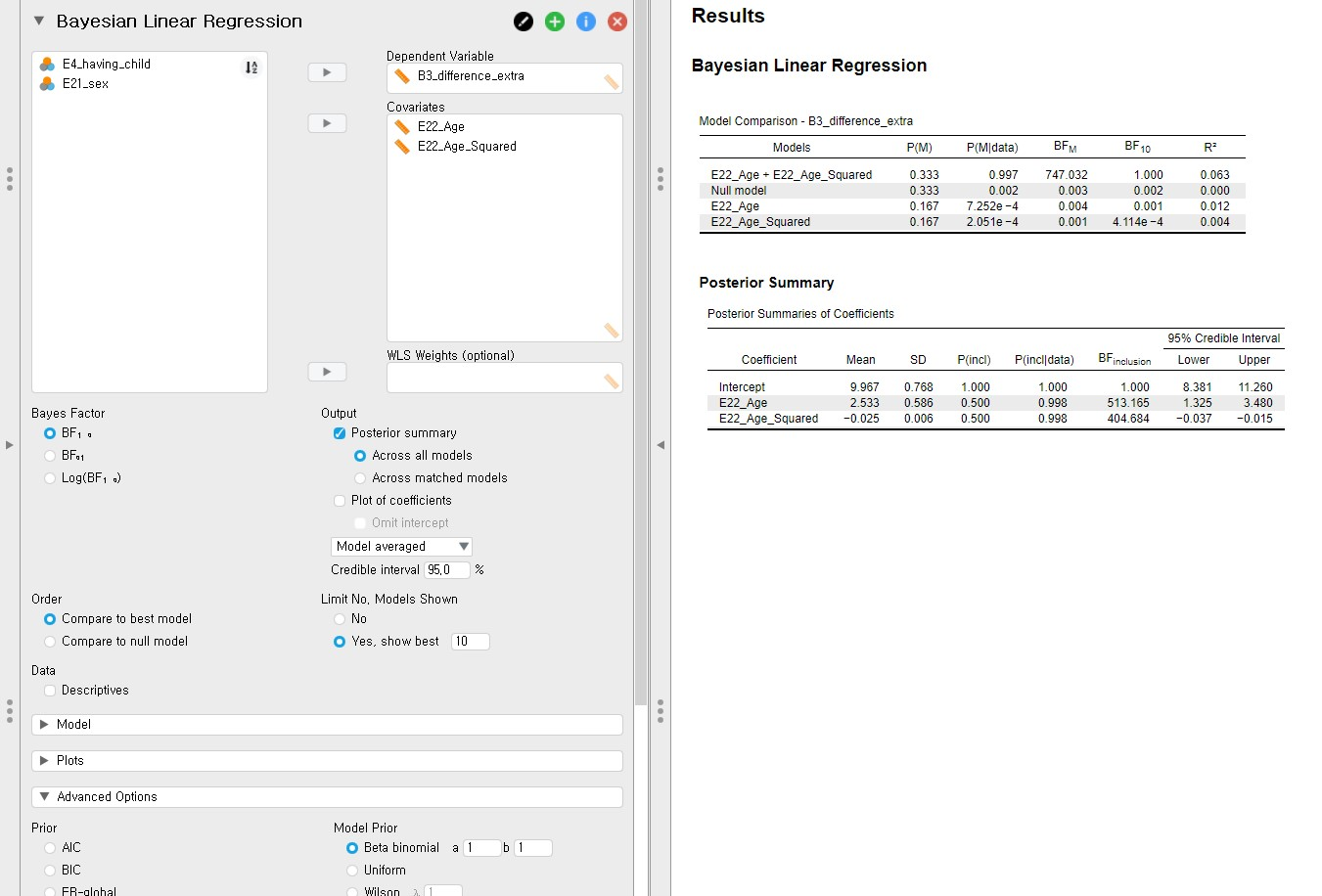• What we have to look at to interpret the results is the Posterior Summaries of Coefficients table in the output panel. The table presents the summary of regression coefficients after taking into account the default priors for age and age-squared variable and the likelihood of the data.
• The posterior mean of the regression coefficient of age is 2.533. We can interpret this value such that a one-year increase in age adds about 2.533 delays in Ph.D. projects on average. The 95% credible interval of age is [1.325, 3.480], which means that there is a 95% probability that the regression coefficient of age lies in the population with the corresponding credible interval. Did you notice that the 95% credible interval does not contain 0? This shows the evidence of the effect of age in predicting the Ph.D. delay.
• The posterior mean of the regression coefficient of age-squared is -0.025. This can be interpreted such that one unit increase of the age-squared variable leads to a decrease of 0.025 unit in the Ph.D. delay on average. The 95% credible interval of [-0.037, -0.015] indicates that we are 95% sure that the regression coefficient of age-squared lies within the corresponding interval in the population. Also, 0 is not included in the interval. Therefore, it is fairly sure that there is an effect of the age-squared variable in predicting the delay.

#### Bayesian model-averaged parameter estimates

• One important fact that we have reserved behind the Posterior Summaries of Coefficients table is that they are model-averaged results of parameter estimation as you can see the word ‘Model averaged’.• To understand what the idea of the model-averaging is, let us recall the regression model that we constructed for the current example: $$Difference = b_{0} + b_{Age} * X_{Age} + b_{Age-squared} * X_{Age-squared}$$
• We based our statistical inference on this single regression model that includes the two predictors, namely age and age-squared. This kind of inference with the single model, however, has inherent risk in the uncertainties of model selection.
• What if the model that does not contain the age variable also provides a good fit to the data and gives substantively different estimates? What if the model that does not contain the age-squared variable is more feasible than the model that contains both predictors after observing the data?
• Consequently, the parameter estimation based on the single model might give misleading results (Hoeting, Madigan, Raftery, & Volinsky, 1999; Hinne, Gronau, Van den Bergh, & Wagenmakers, 2020; Van den Bergh et al., 2020). Bayesian model averaging can solve this issue such that one can get parameter estimates after considering all the candidate models (i.e., the number of models that one can construct given the predictors).
• Okay, this sounds like a great idea. However, how do we average estimates across the candidate models? The answer is to average estimates based on the posterior model probabilities. In Bayesian statistics, the probability of the model given the observed data is called the posterior model probability, which is interpreted as the relative probability in favor of the model under consideration after observing the data.
• Then, how do we know how probable each candidate model is before seeing the data? The reply is to assign the prior model probabilities to each candidate model. Prior model probability is the relative plausibility of the models under consideration before observing data. In other words, prior model probability tells us how probable the model is before we see data.
• In JASP, we can set the prior model probability in the Model Prior column under the Advanced Options section. By default, the Beta binomial distribution with a = 1 and b = 1 is chosen. Although we proceeded with this setting, researchers can choose other options. For example, the researchers can assume that all models are equally likely by selecting the Uniform model prior. This option is a neutral choice, as Hoeting, Madigan, Raftery, and Volinsky illustrated (1999).
• In our example where there are two predictors, there are four candidate models: the model that does not contain any predictors (also called the null model), the model that only contains the age variable, the model that only contains the age-squared variable, and the model that contains both predictors. The JASP provides these the candidate models in the output panel. Where? The table titled Model Comparison – B3_difference_extra is the one! Therefore, the Posterior Summaries of Coefficients table provides the parameter estimates after taking into account all the candidate models in the Model Comparison table.

#### Inclusion Bayes factor

• At this point, we would like to introduce the concept of the inclusion Bayes factor ($$BF_{inclusion}$$), one of the columns in the Posterior Summaries of Coefficients table.
• The inclusion Bayes factor quantifies how much the observed data are more probable under models that include a particular predictor relative to the models that do not contain that particular predictor.
• For the age variable, the inclusion Bayes factor is 513.165. We can interpret this value such that, across all the candidate models, the model with the age variable is, on average, about 513 times more likely than the model without the age variable.
• For the age-squared variable, the inclusion Bayes factor is 404.684. This implies that the model that contains the age-squared variable is, on average, about 405 times more likely than the model without the age-squared variable considering all the candidate models.

#### Parameter estimates for the best model

• You might want to investigate the parameter estimates under the best single model that is the most probable given the observed data. We only need to change the ‘Model averaged’ option into the ‘Best model’ option.
• The interpretation of the Posterior Summaries of Coefficients table is the same as above except for the fact that now the estimates are not averaged but from the best model.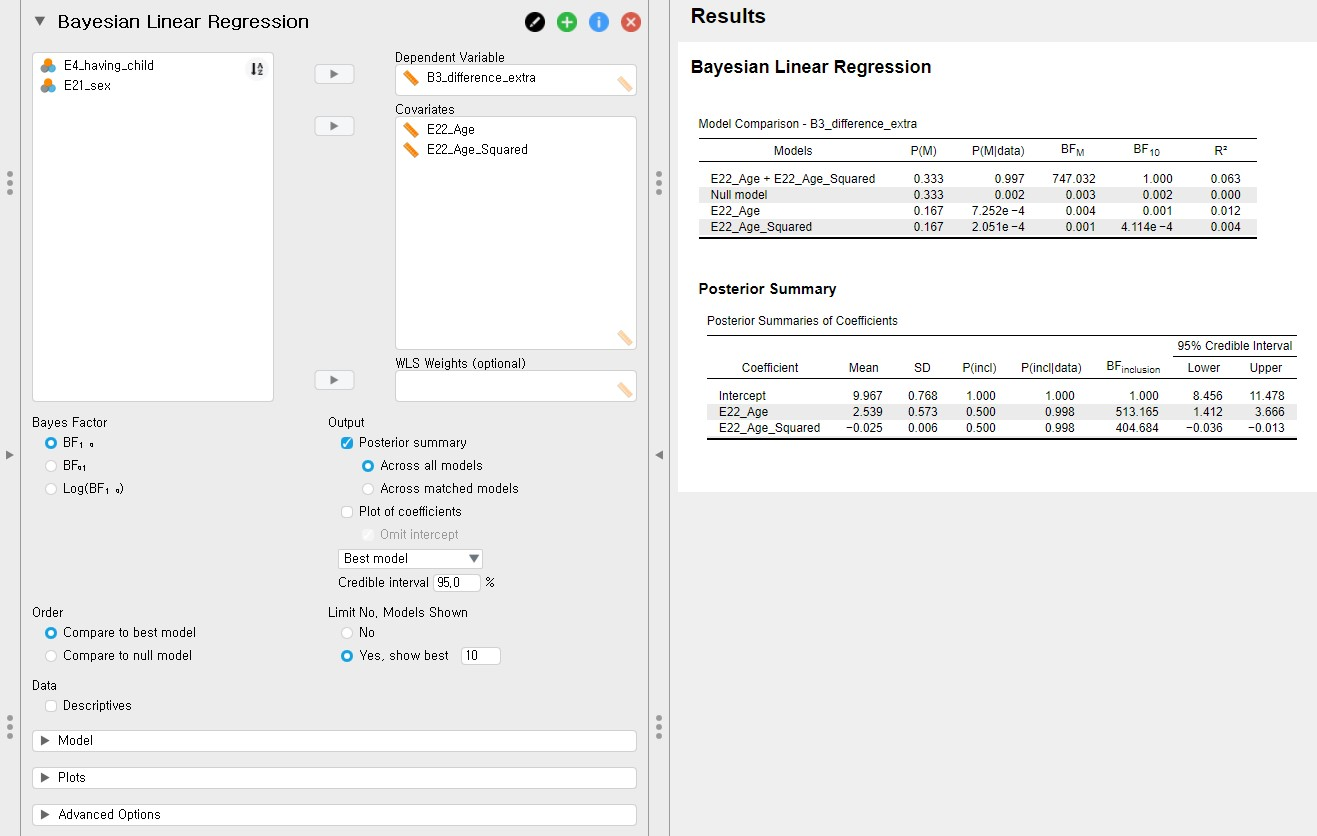• “What is the best model, in this case?” This is a very good question! The best model is the most probable or feasible model after observing the data. To choose that model, the probability of the model given the observed data (i.e., the posterior model probability) should be the highest.
• In the Model Comparison table, the column of P(M|data) denotes the posterior model probability. In our example, the model that contains both predictors has the highest posterior model probability, which is 0.997 (almost 1). What is P(M), then? It is the prior model probability that we have explained previously.
• According to the Model Comparison table, for the regression model that contains both predictors (i.e., E22_Age + E22_Age_Squared), the probability of the model has increased from 33.3% to 99.7%, after observing the model.
• The $$R^{2}$$ we see in the last column of the Model Comparison table is the proportion of variance explained by the predictors in the model. The model that contains both predictors has the highest $$R^{2}$$ of 0.063 among all candidate models. The $$R^{2}$$ of 0.063 means that the age and age-squared variable explains 6.3% of the variance in the Ph.D. delay.
• For more information about the model-averaged parameter estimates and the interpretation of the tables in JASP, we refer readers to Van den Bergh and others (2020).
• In the upcoming sections, we keep using the model-averaged estimates.

### 2. A graphical examination for posterior summaries

• For the Posterior Summary in the output panel, we can take a look at the same information visually. This way enables us to understand the results with more intuition.

#### Plot of coefficients

• Among many numbers from the Posterior Summaries of Coefficients, we are primarily keen on the posterior mean and the 95% credible interval of parameters. Plot of coefficients serves the purpose that helps us out.
• Check Plot of coefficients in the control panel -> Check Omit intercept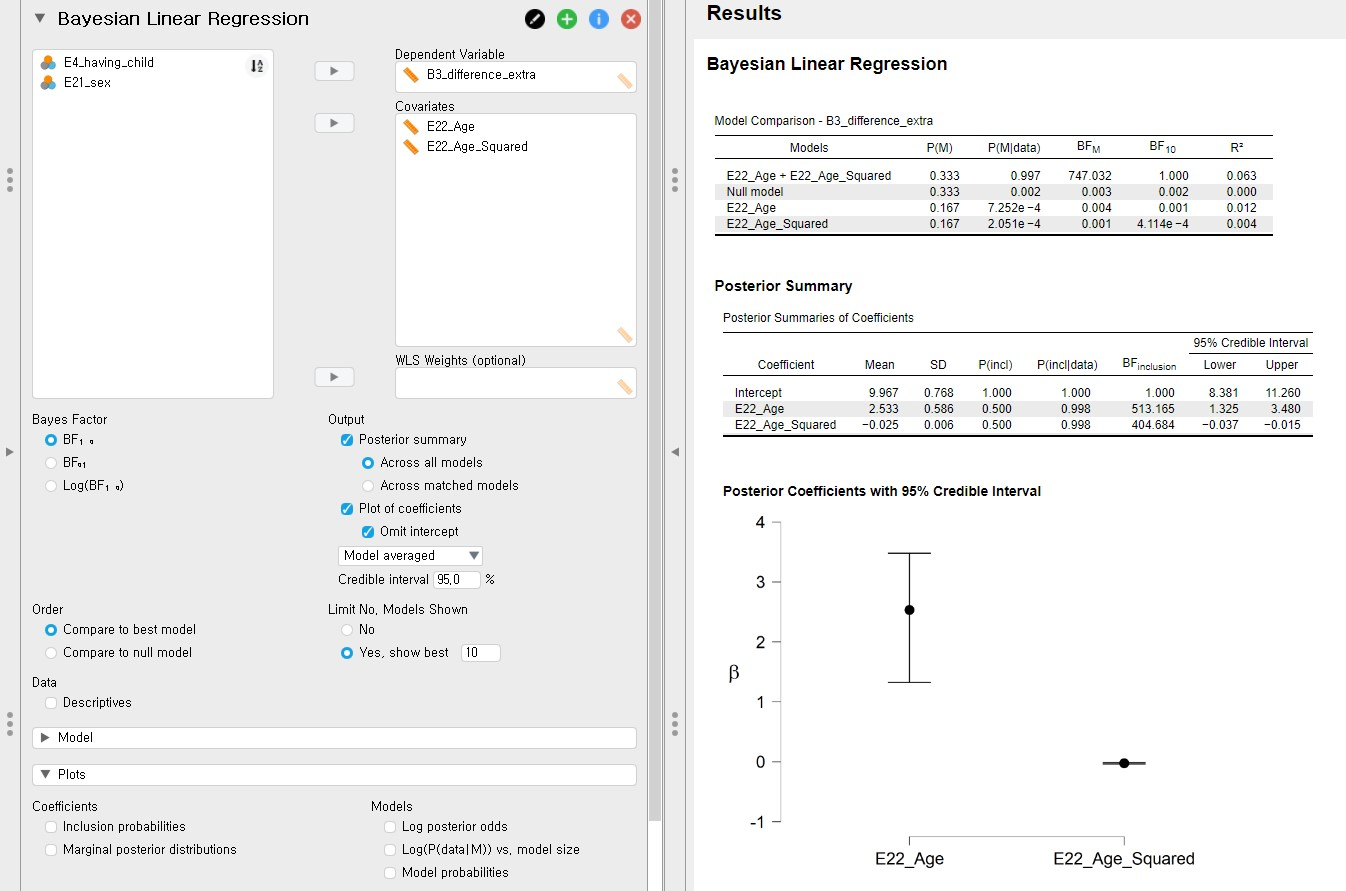• In the output panel, you can see the Posterior Coefficients with 95% Credible Interval. This plot shows the posterior distributions of both regression coefficients in the current model.
• The black round dot corresponds to the posterior mean of each regression coefficient. The upper and lower whisker surrounds the 95% credible interval. For the age variable, the 95% credible interval does not include 0. For the age-squared variable, it is difficult to clearly see whether the 0 is included in the 95% credible interval since the interval is very narrow and close to 0. In this case, we should refer to the numbers in the Posterior Summaries of Coefficients table.
• “Why did we decide to omit the intercept in the plot?” This is a great question! In our regression example, the intercept means the average of the Ph.D. delay when the values of age and age-squared are zero. We previously saw from the descriptive statistics that the mean and the standard error of the mean for the age variable are 31.68 and 0.38. Therefore, interpreting the meaning of intercept in this context is not meaningful. Rather, plotting the posterior distributions for the regression coefficients of age and age-squared is more informative.

#### Marginal posterior distribution

• What if we want to see the posterior density of each parameter? Drawing the marginal posterior distribution is the one that solves our thirst.
• Click Plots in the control panel -> Check Marginal posterior distributions under the Coefficients section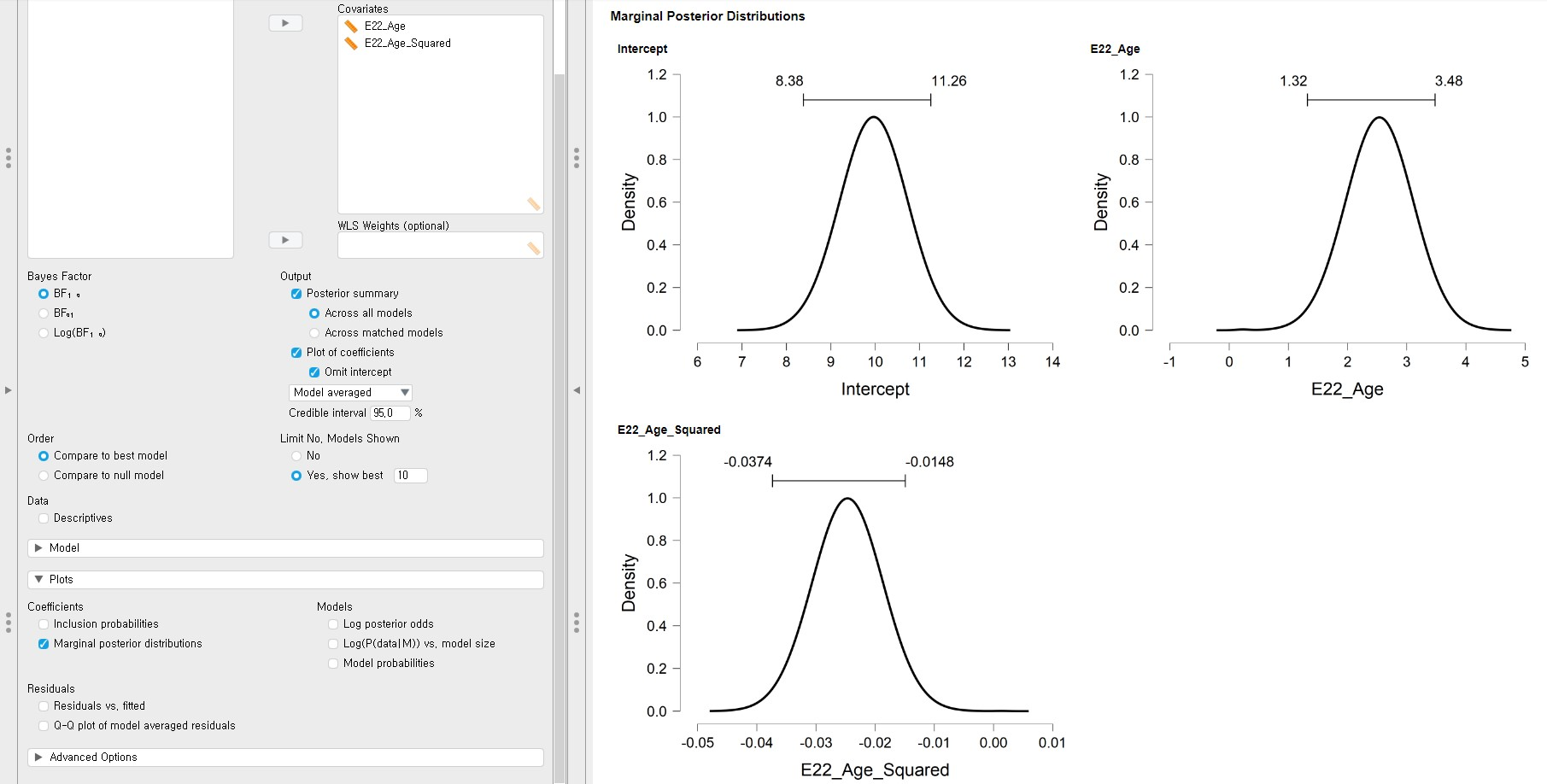• Again, we only focus on the marginal posterior distributions of age and age-squared variable. The bar above the highest peak corresponds to the 95% credible interval with the lower limit on the left and upper limit on the right. This interval is the same as the 95% credible interval in the Posterior Summaries of Coefficients table.

### 3. So, what is the default prior for the regression coefficients?

• Behind what we have done in interpreting the results, the default prior is hiding. To seek the default prior, click Advanced Options in the control panel. The left column, named Prior, presents the priors we can take.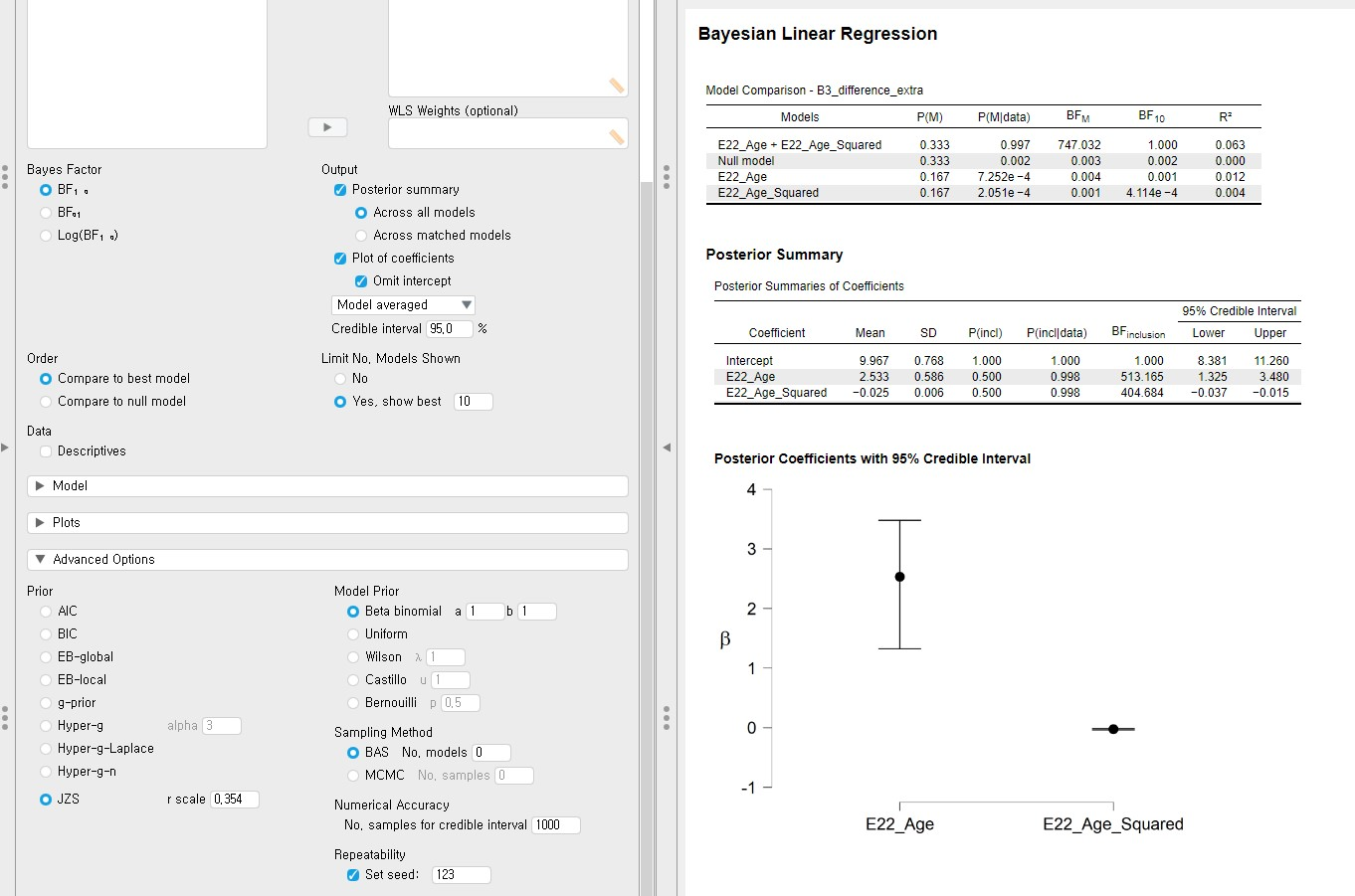• By default, the JZS prior with an r scale of 0.354 is chosen. The JZS prior stands for the Jeffreys-Zellner-Siow prior. Yes, it is the default prior that JASP uses for Bayesian regression!
• Technically speaking, the JZS prior assigns the normal distribution to each regression coefficient (Andraszewicz et al., 2015). The JASP provides other options for prior choices (AIC, BIC, EB-global, EB-local, g-prior, Hyper-g, Hyper-g-Laplace, Hyper-g-n). The JZS prior, however, is most recommended and advocated as the default prior when performing the Bayesian regression analysis. Therefore, we proceed with adjusting the r scale values of the JZS prior. For readers who want to understand the aforementioned priors, see Liang, Paulo, Molina, Clyde, and Berger (2008).
• Please note that under the Advanced Options section, the Prior column on the left is about setting the prior distributions on the regression parameters whereas the Model Prior column on the right is assigning prior model probabilities.

## V. Changing the r scale values of the JZS prior

• By setting different values of r scale instead of 0.354, we can change the scale or variance but not the location of the JZS prior. This has something to do with what is called the prior sensitivity.
• You might wonder how to incorporate prior knowledge by using different prior distributions and adjusting hyperparameters of them. We cannot do this in the default Bayesian Linear Regression option in the JASP (Version 0.13.1). However, incorporating prior knowledge, hence using informative priors, is possible through using Jags. In ‘VI. Ready for action’, we will guide you to the tutorial that explains how to do that.
• The prior sensitivity, in a nutshell, tells us how robust the estimates are depending on the width of the prior distribution. Although we do not discuss the topic of prior sensitivity in detail, it is good to remember the key idea in case you encounter the concept in the Bayesian literature (for interested readers on the idea of prior sensitivity, see Van Erp, Mulder, and Oberski, 2018).
• In JASP, changing the r scale values specifically indicates how certain we are about the null effects of parameters in the regression analysis. Larger values for the r scale correspond to wider priors whereas smaller values lead to the narrower priors.
• If a wider prior is adopted, hence more spread-out the prior distribution is, we are unsure about the effect of parameters. A narrower prior, on the other hand, means we have a strong belief that the effect is concentrated near zero (i.e., the null effect).
• The focus here is to compare the results of different r scale specifications (0.001, 0.1, 10, and 1000) of the JZS prior. Let’s see what happens in the parameter estimates and the inclusion Bayes factors of the Posterior Summaries of Coefficients table and the marginal posterior distributions.
• In the marginal posterior distributions, you will encounter the grey spike at zero with its height. The spike means the absence of the effect of the regression coefficient. The height indicates how much the data favors the exclusion of a specific predictor in the regression model after observing the data. This height is also called the posterior exclusion probability. If the posterior exclusion probability is high, this means that the observed data does not support to include the predictor.

### 1. Adjusting the r scale values (0.001, 0.1, 10, and 1000)

#### JZS prior with the r scale of 0.001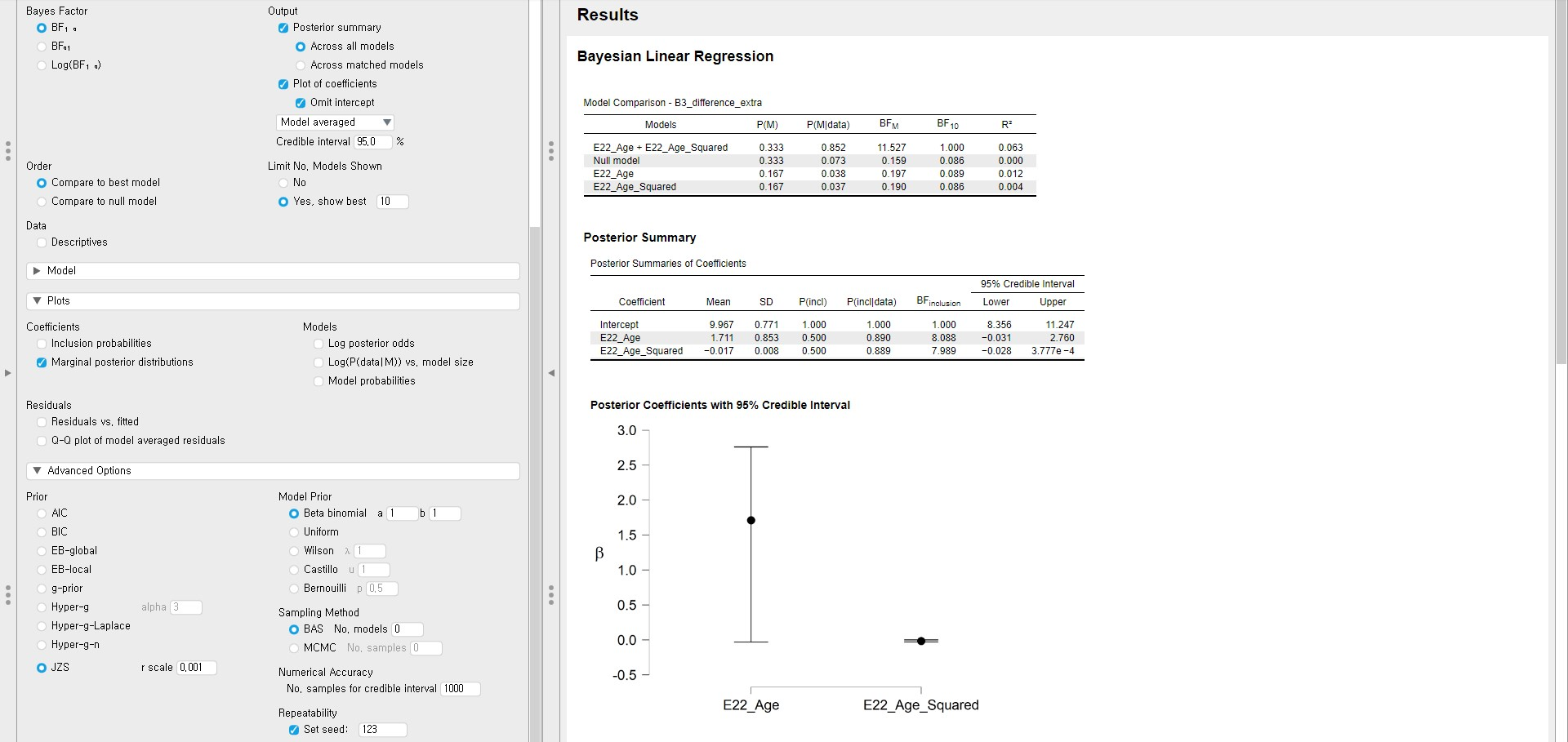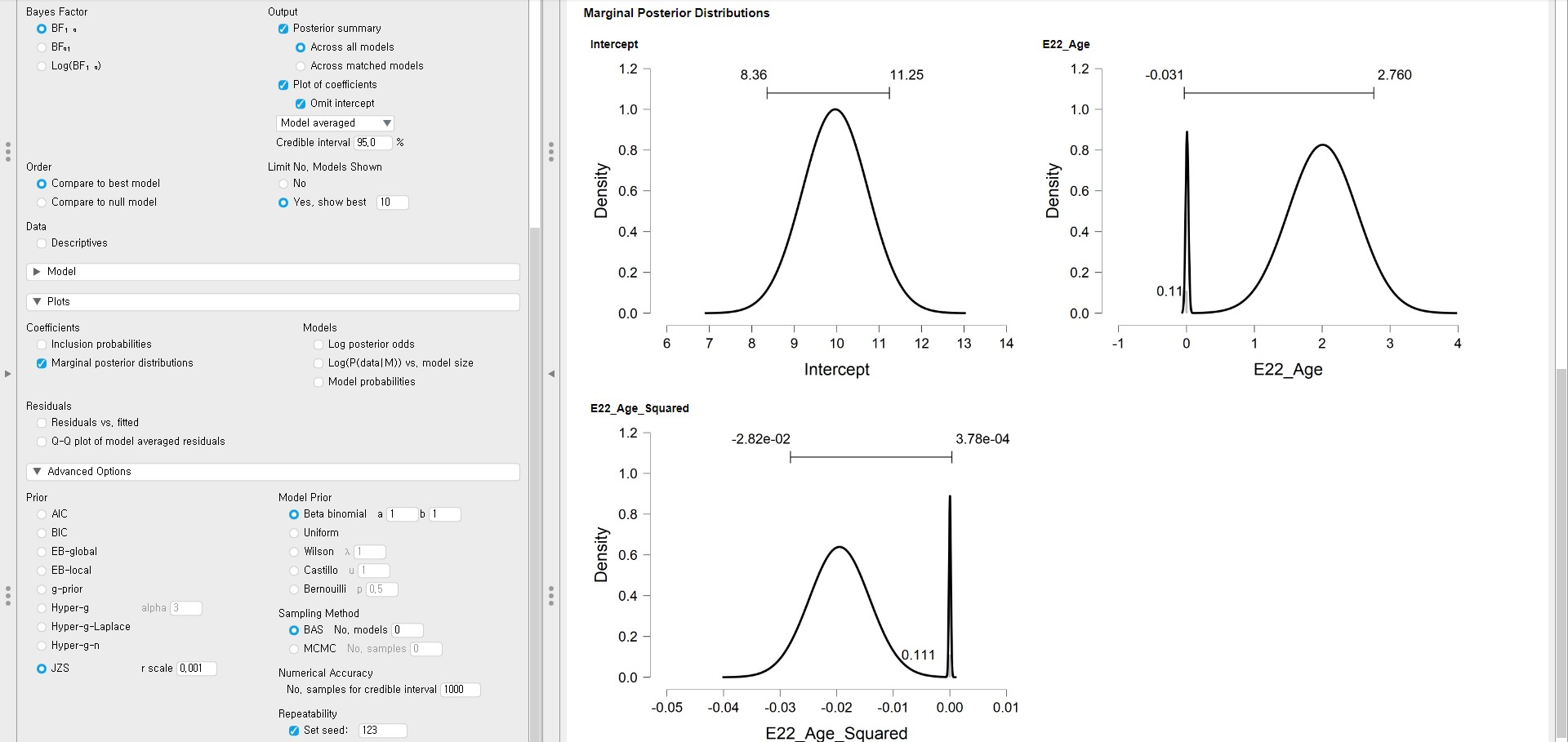#### JZS prior with the r scale of 0.1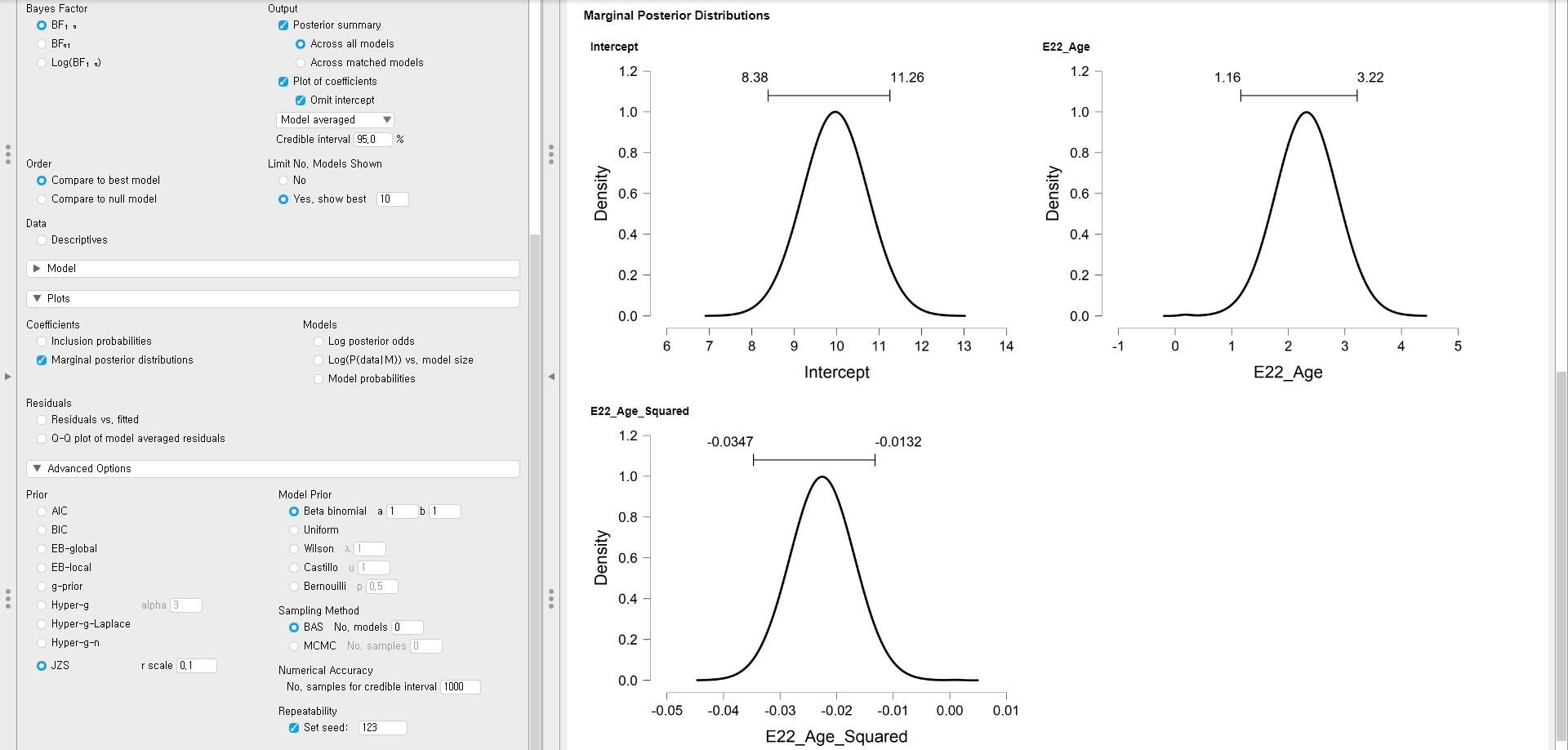#### JZS prior with the r scale of 10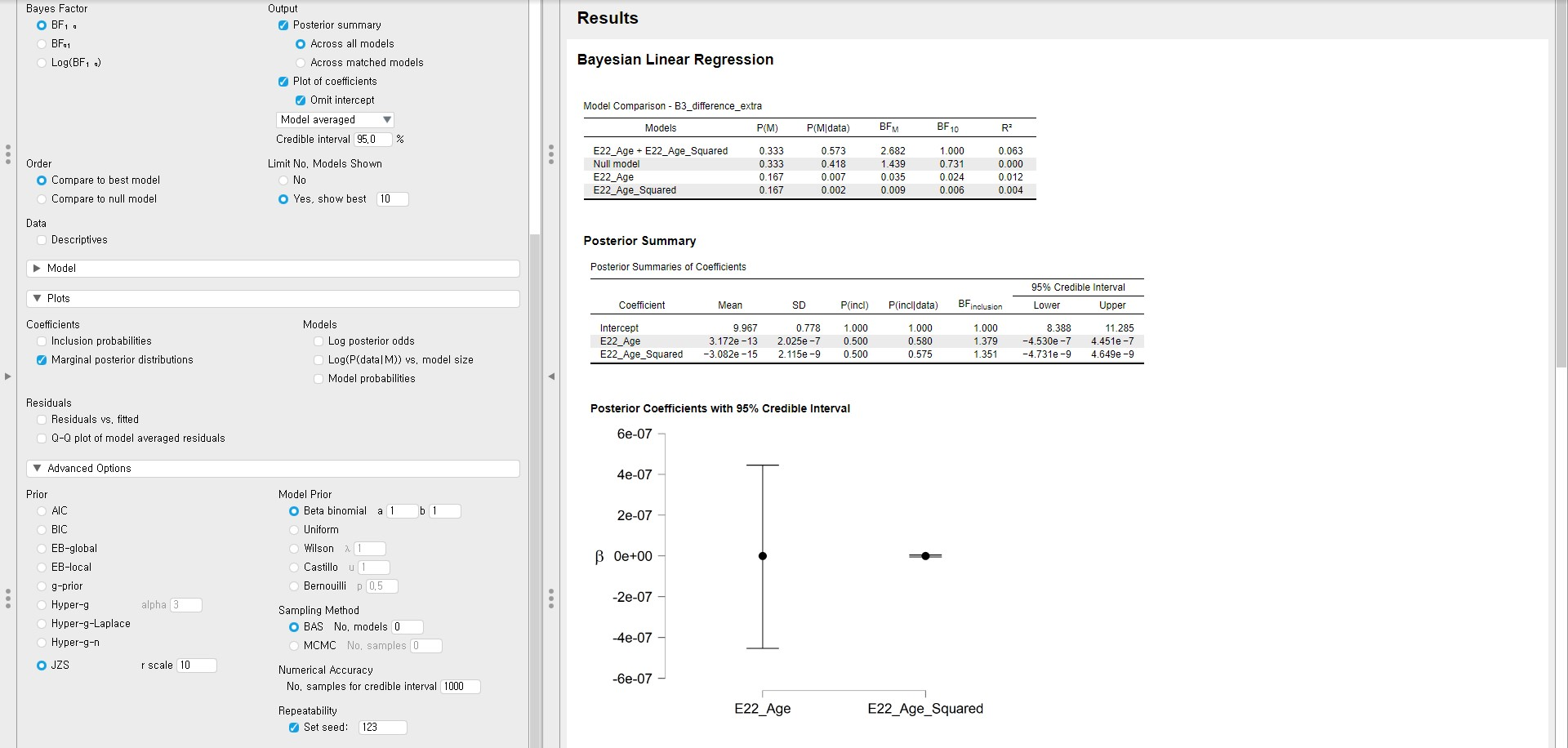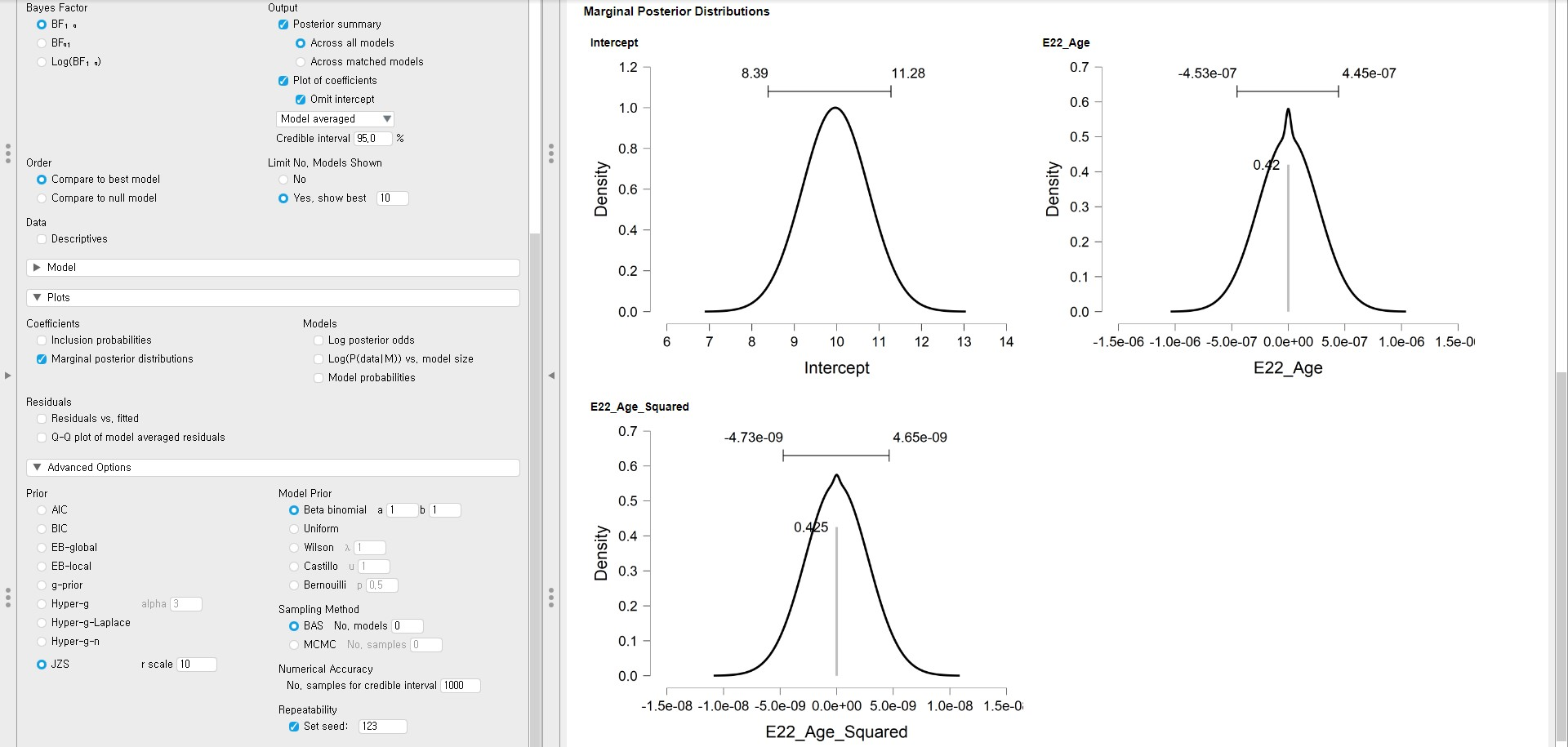#### JZS prior with the r scale of 1000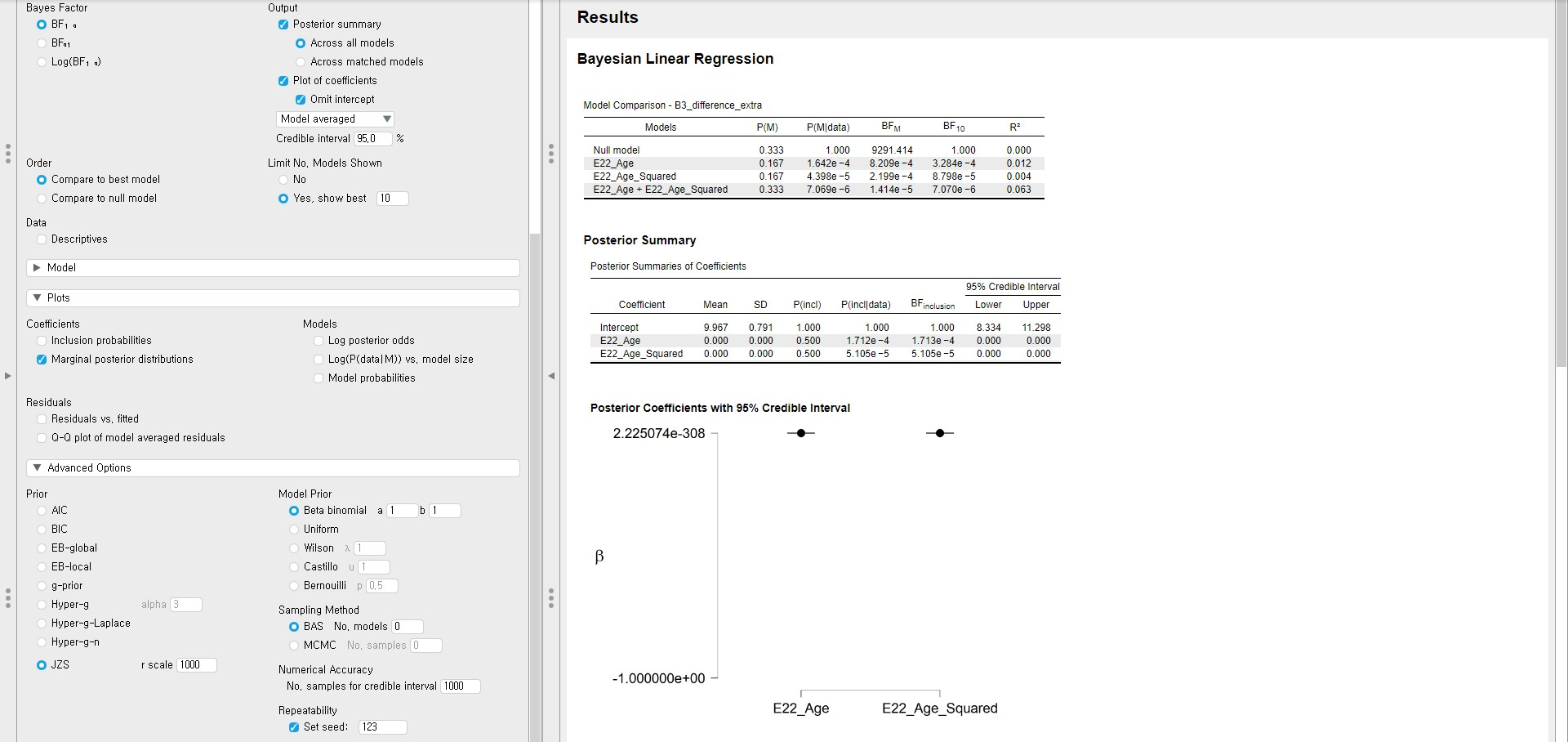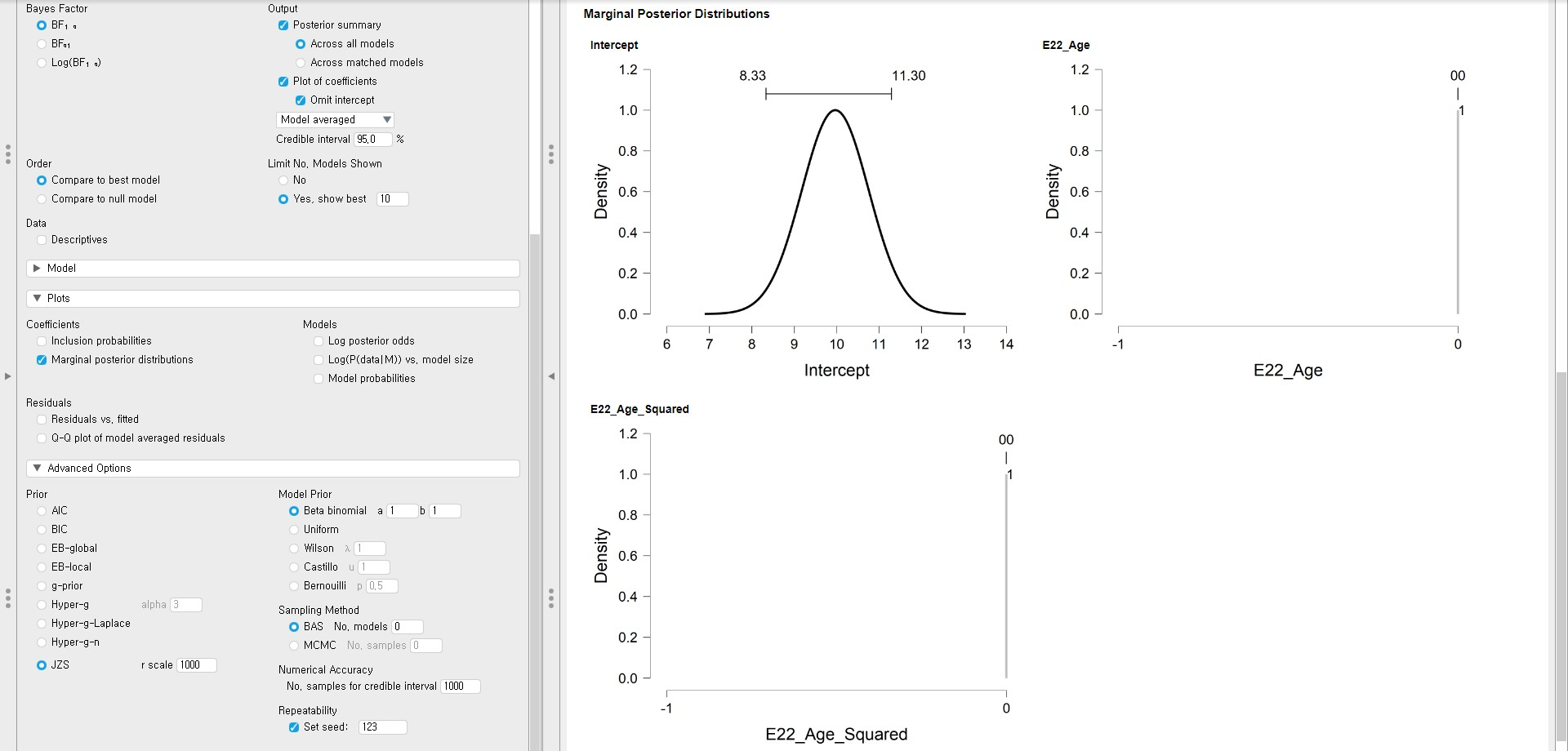#### Summary of the posterior mean and the posterior standard deviation

Age Default (JZS with r = 0.354) JZS with r = 0.001 JZS with r = 0.1 JZS with r = 10 JZS with r = 1000
Posterior mean 2.533 1.711 2.315 3.172e-13 0.000
Posterior standard deviation 0.586 0.853 0.560 2.025e-7 0.000

Age-squared Default (JZS with r = 0.354) JZS with r = 0.001 JZS with r = 0.1 JZS with r = 10 JZS with r = 1000
Posterior mean -0.025 -0.017 -0.022 -3.082e-15 0.000
Posterior standard deviation 0.006 0.008 0.006 2.115e-9 0.000

### 2. Effect of different prior specifications on parameter estimation

• Can you compare the results of parameter estimation over the different prior specifications? To examine whether the results are comparable with the analysis with the default prior, we check the two things: the relative bias and the change of parameter estimates from the Posterior Summaries of Coefficients table.

#### Relative bias

• The relative bias is used to express the difference between the default prior and the user-specified prior.
• The formula for the relative bias follows: $$bias = 100*\frac{(model \; with \; specified \; priors\; – \; model \; with \; default \;priors)}{model \; with \; default \; priors}$$
• To preserve clarity, we will just calculate the bias of the two regression coefficients and only compare the model with the default prior (JZS prior with the r scale of 0.354) and the model with the different r scale value (JZS prior with the r scale of 0.001).
• For the age variable, the relative bias is $$100*\frac{(1.711\; – \; 2.533)}{2.533}$$. This equals -32.45%.
• For the age-squared variable, the relative bias is $$100*\frac{(-0.017 \; – \; (-0.025))}{-0.025}$$, which equals -32%.
• We see that the influence of the user-specified prior is around -32% for both regression coefficients. This is a sign of change in the results with different prior specifications. For the concrete examination, let’s take a look at the parameter estimates.

#### Parameter estimates

$$Age$$ Default (JZS with r = 0.354) User-specified (JZS with r = 0.001)
Posterior mean 2.533 1.711
Posterior standard deviation 0.586 0.853
95% credible interval [1.325, 3.480] [-0.031, 2.760]

$$Age^{2}$$ Default (JZS with r = 0.354) User-specified (JZS with r = 0.001)
Posterior mean -0.025 -0.017
Posterior standard deviation 0.006 0.008
95% credible interval [-0.037, -0.015] [-0.028, 3.777e-4]

• For both variables, there are changes in the parameter estimates. Specifically, the 95% credible intervals with the default prior do not include 0. However, 0 is included in the 95% credible intervals when the user-specified prior is used. Therefore, there is no evidence of the effect of regression coefficients when we use the prior with the r scale of 0.001. These results are understandable since we set the very small r scale value (0.001), which indicates our strong belief that there is no effect of predictors.

### 3. Effect of different prior specifications on inclusion Bayes factor

• This time, let’s see how the inclusion Bayes factor changes over the different prior specifications. We again check the relative bias and the change of inclusion Bayes factors from the Posterior Summaries of Coefficients table.

#### Relative bias

• We compute the bias of the inclusion Bayes factors of the two regression coefficients and only compare the model with the default prior (JZS prior with the r scale of 0.354) and the model with the different r scale value (JZS prior with the r scale of 0.001).
• For the age variable, the relative bias is $$100*\frac{(8.088\; – \; 513.165)}{513.165}$$. This equals -98.42%.
• For the age-squared variable, the relative bias is $$100*\frac{(7.989\; – \; 404.684)}{404.684}$$, which equals -98.03%.
• We see that the influence of the different prior specifications is around -98% for both inclusion Bayes factors. This is a sign of a large change in the results with different prior specifications. For the concrete examination, let’s take a look at the values of the inclusion Bayes factor.

#### Inclusion Bayes factor

$$Age$$ Default (JZS with r = 0.354) User-specified (JZS with r = 0.001)
$$BF_{inclusion}$$ 513.165 8.088

$$Age^{2}$$ Default (JZS with r = 0.354) User-specified (JZS with r = 0.001)
$$BF_{inclusion}$$ 404.684 7.989

• For both variables, the inclusion Bayes factors with the r scale of 0.001 are much smaller than that with the default prior. This happened because a strong belief about the null effect of the regression coefficients is reflected in the smaller r scale value.

### 4. Conclusion about the effect of different prior specifications

• Given the relative bias and the values of the parameter estimates and the inclusion Bayes factor, we conclude there is a difference from different prior specifications. Therefore, we would not end up with similar conclusions.
• The influence of prior also depends on the size of the dataset. The sample size for our data is 333, which is relatively big. In the case of the big dataset, the influence of the prior is relatively small. If one would use a small dataset, on the other hand, the influence of the prior becomes larger.